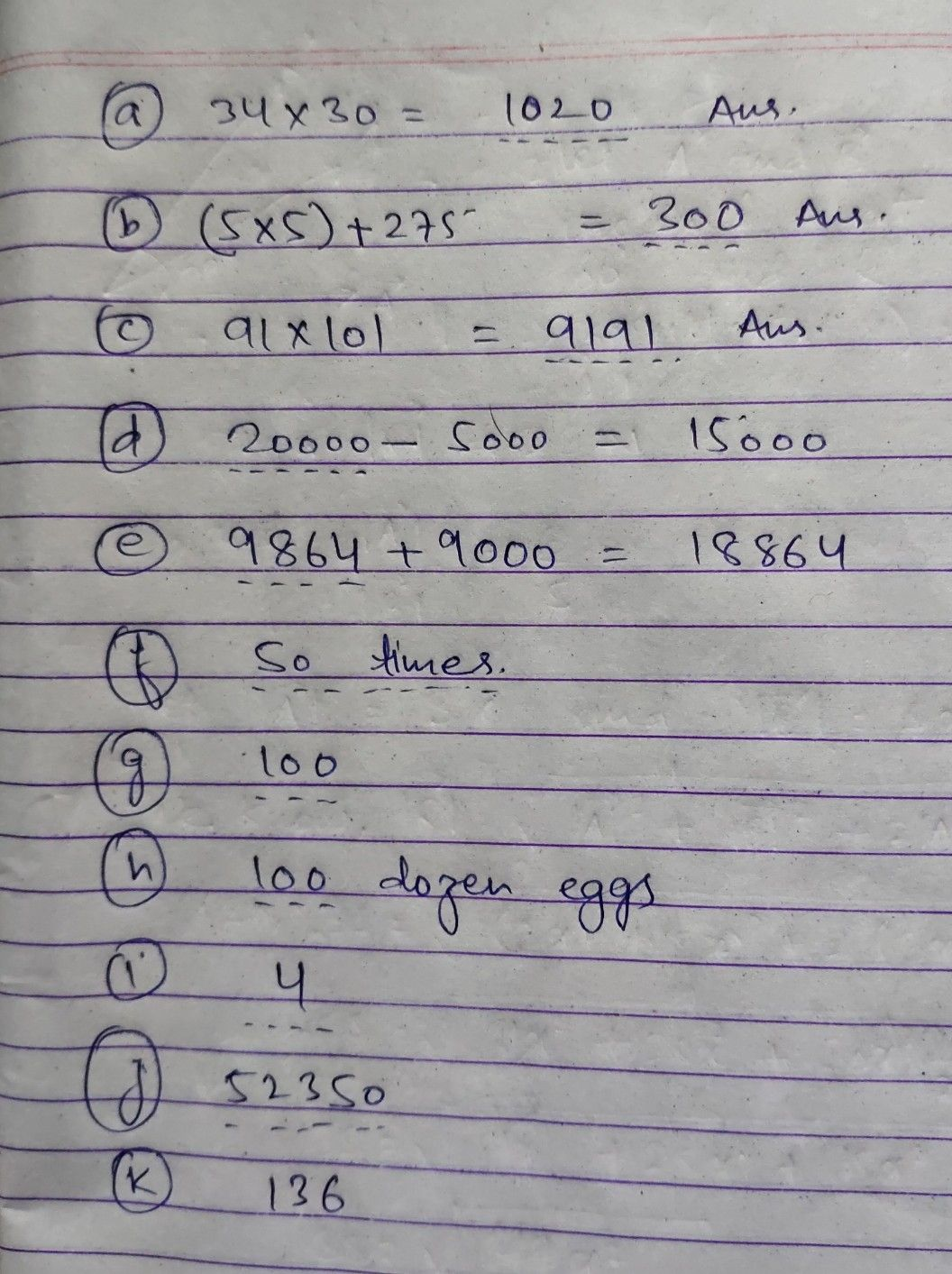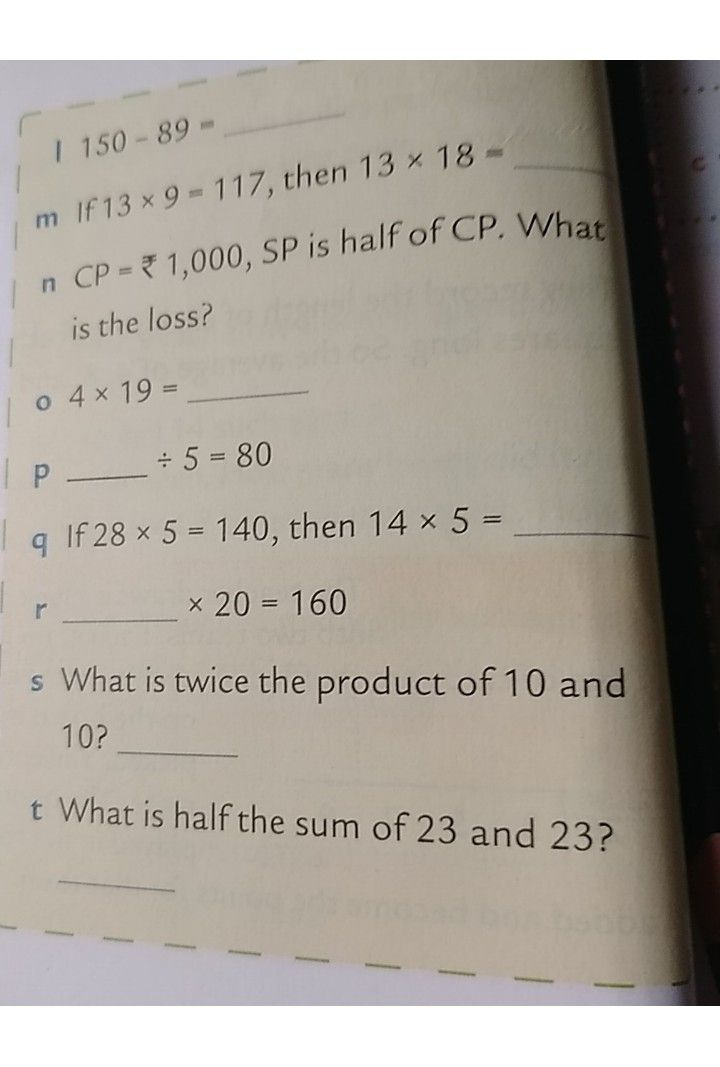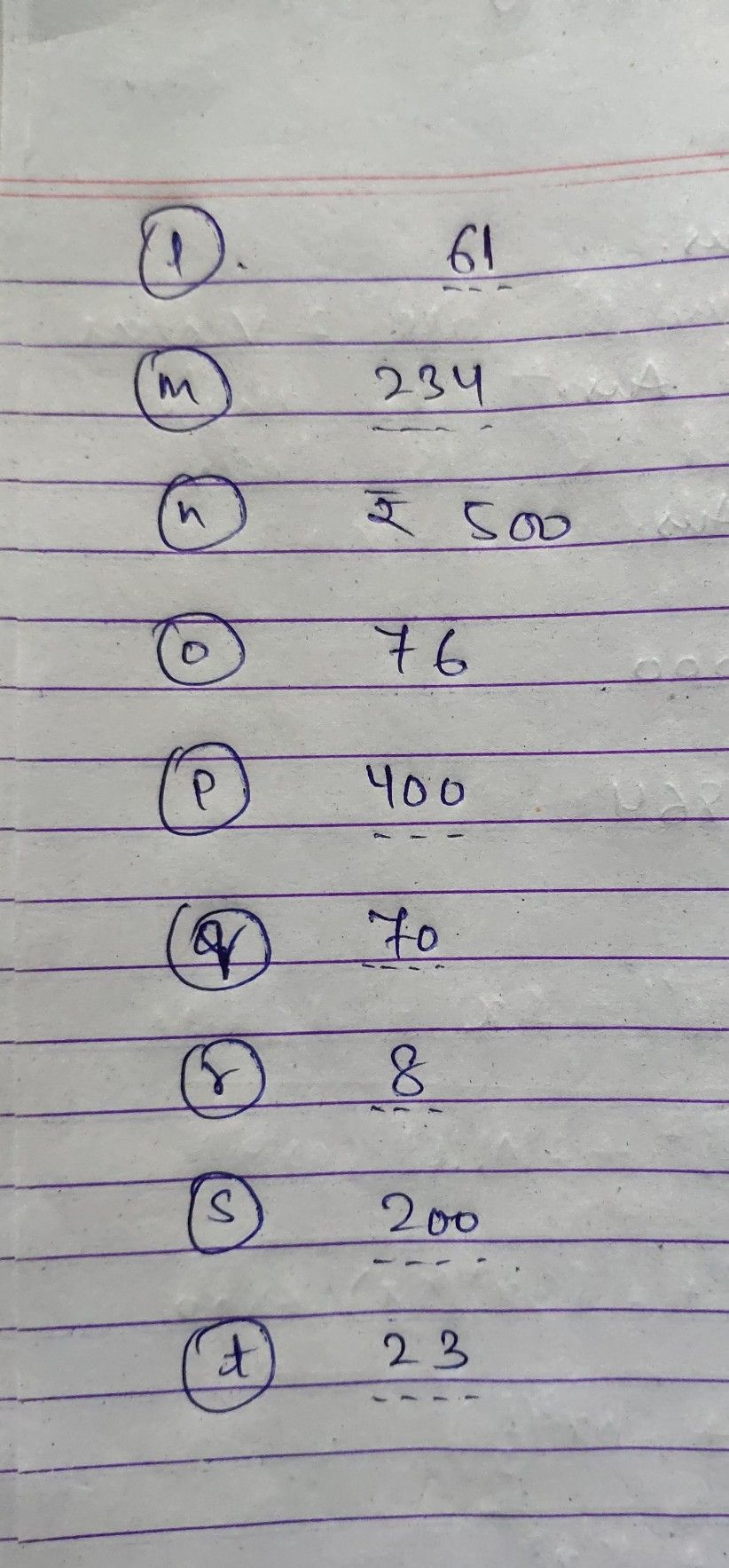Symbol
Problema $34\times 30=$ b Add the product of 5 and 5 to $275.$ $c$ $c91\times 101=$ $-$ $1$ d $-5000=15000$ e $+9000=18864$ f How many times $50$ $is$ $2,5002$ g $5000\div 50=$ h $1200$ $eggs=$ dozen eggs i How many $6sin$ $6,6667$ $58350-6000=$ $k97+39=$